# RS Aggarwal Solutions for Class 9 Chapter 4: Linear Equations in Two Variables

The equations which contain two variables and can be represented as ax + by = c where a, b and c are constants is called linear equations in two variables. Various methods like elimination method, substitution method and graphing method can be made use of in order to solve the linear equations in two variables.

The students can make use of the RS Aggarwal Solutions for Class 9 which is a good reference material according to the syllabus. RS Aggarwal Solutions for Class 9 Chapter 4 Linear Equations in Two Variables are provided here.

## RS Aggarwal Solutions for Class 9 Chapter 4 : Linear Equations in Two Variables Download PDF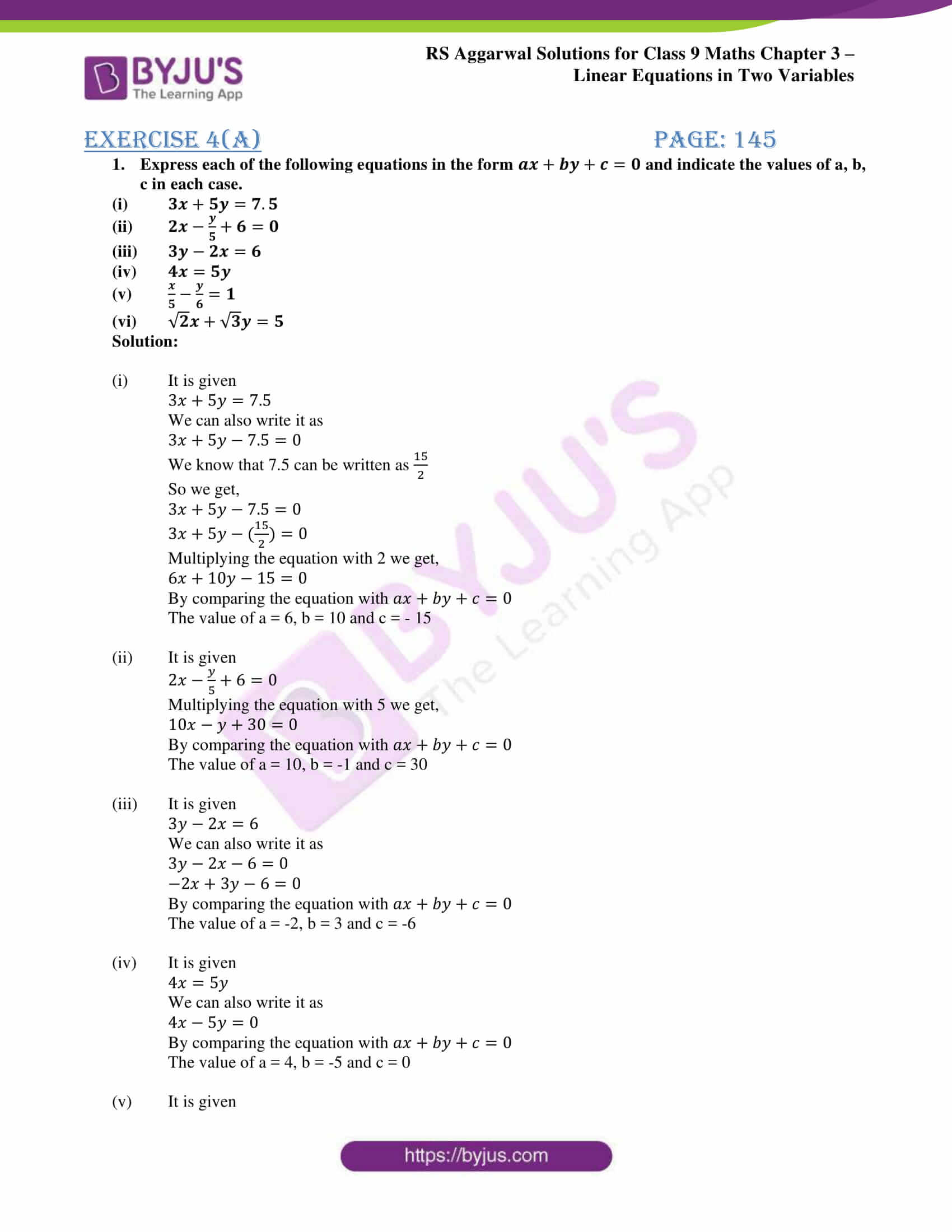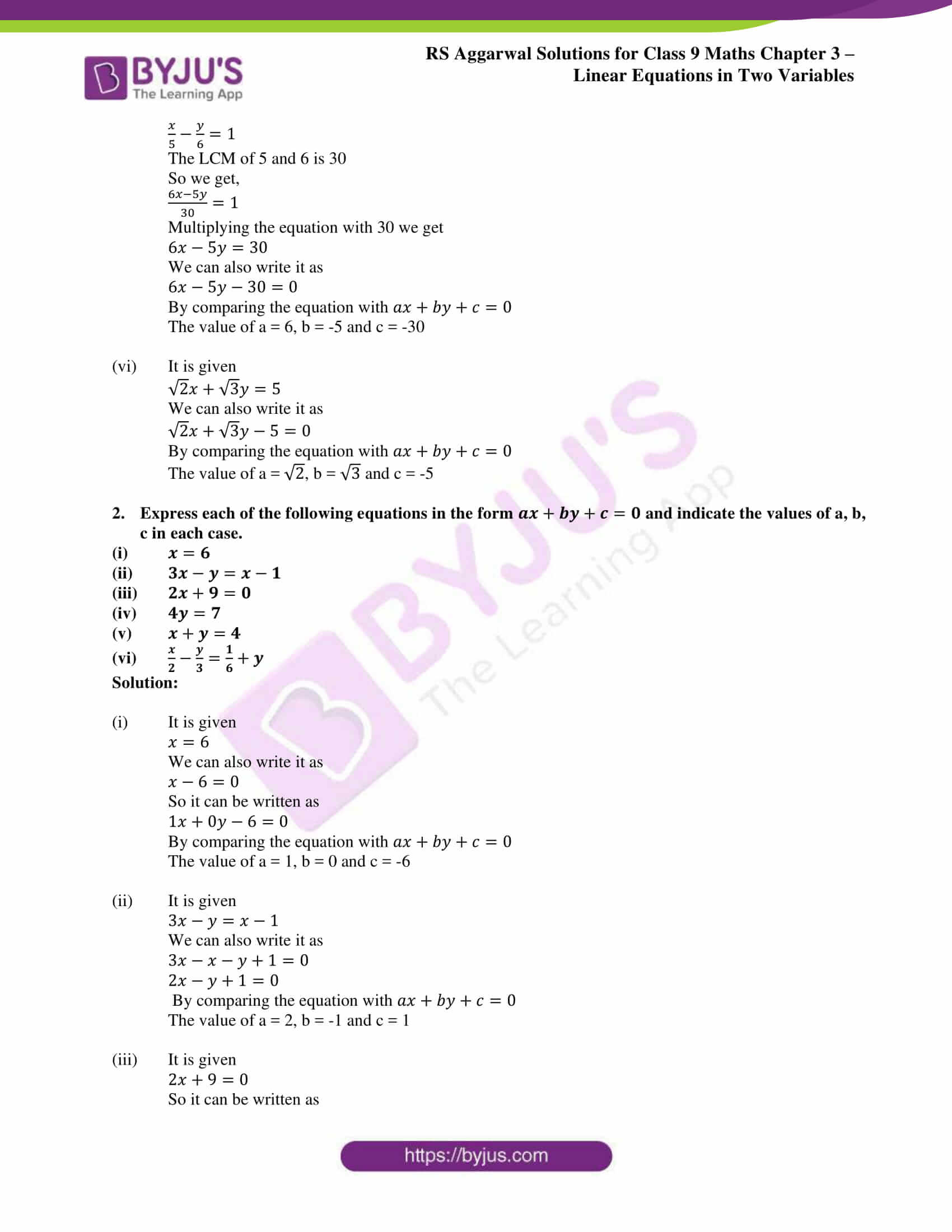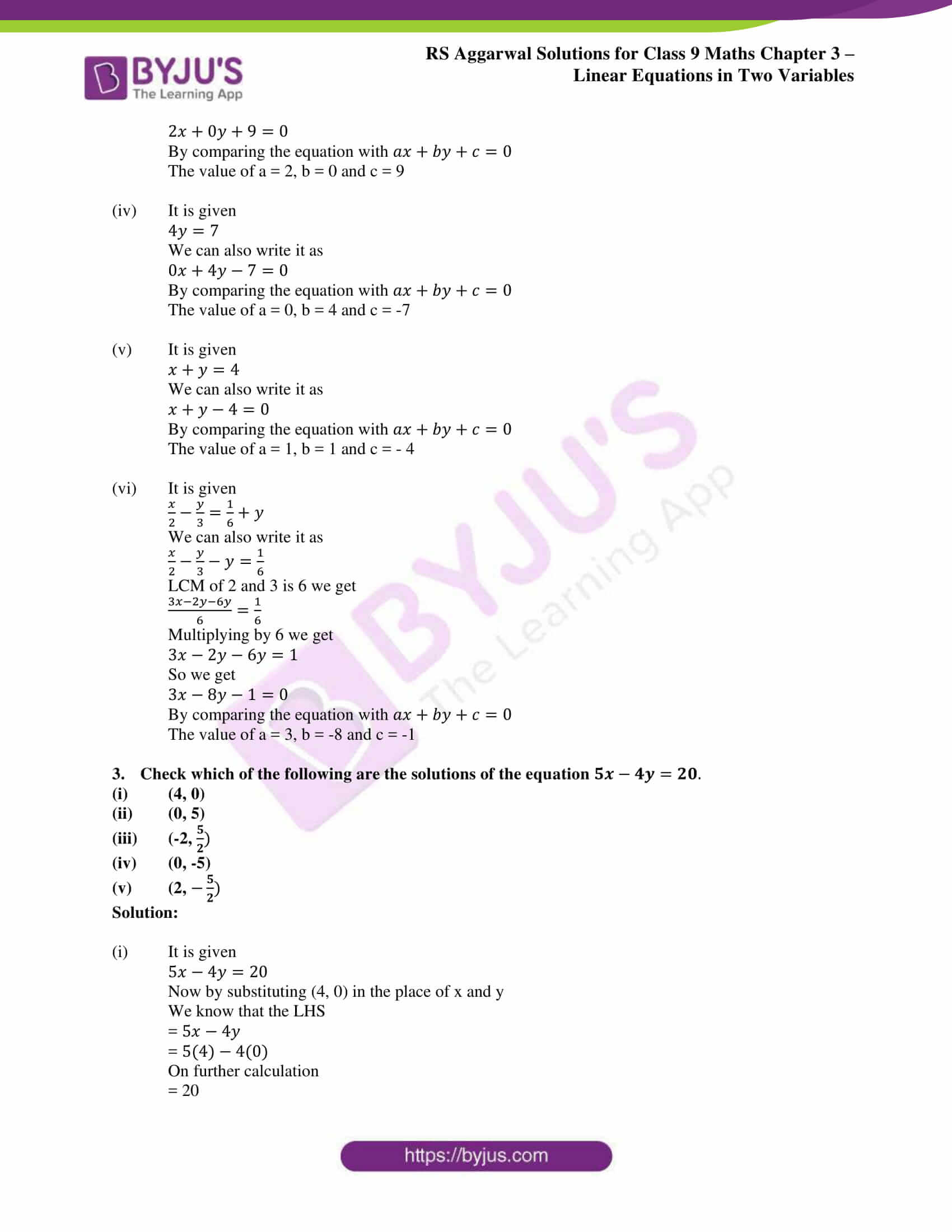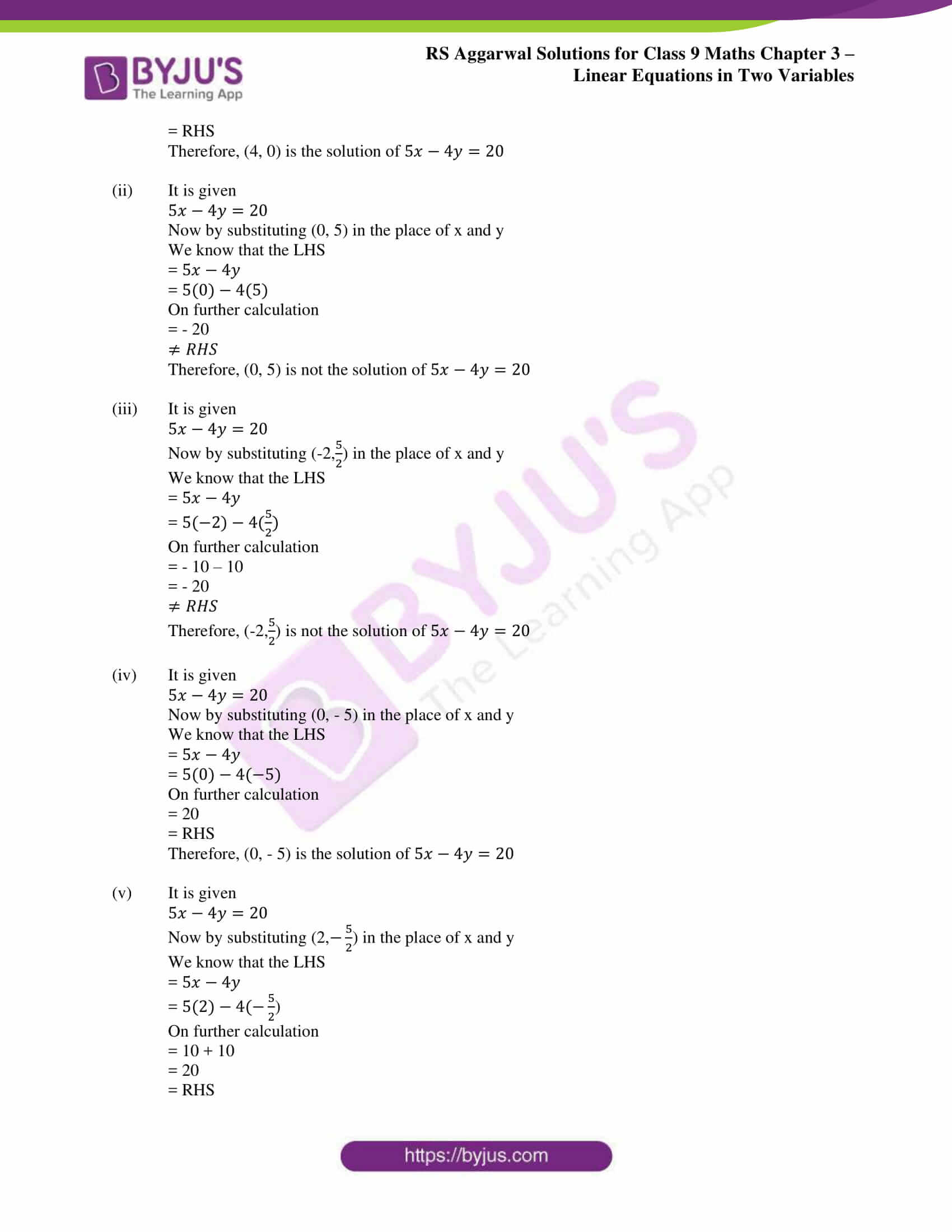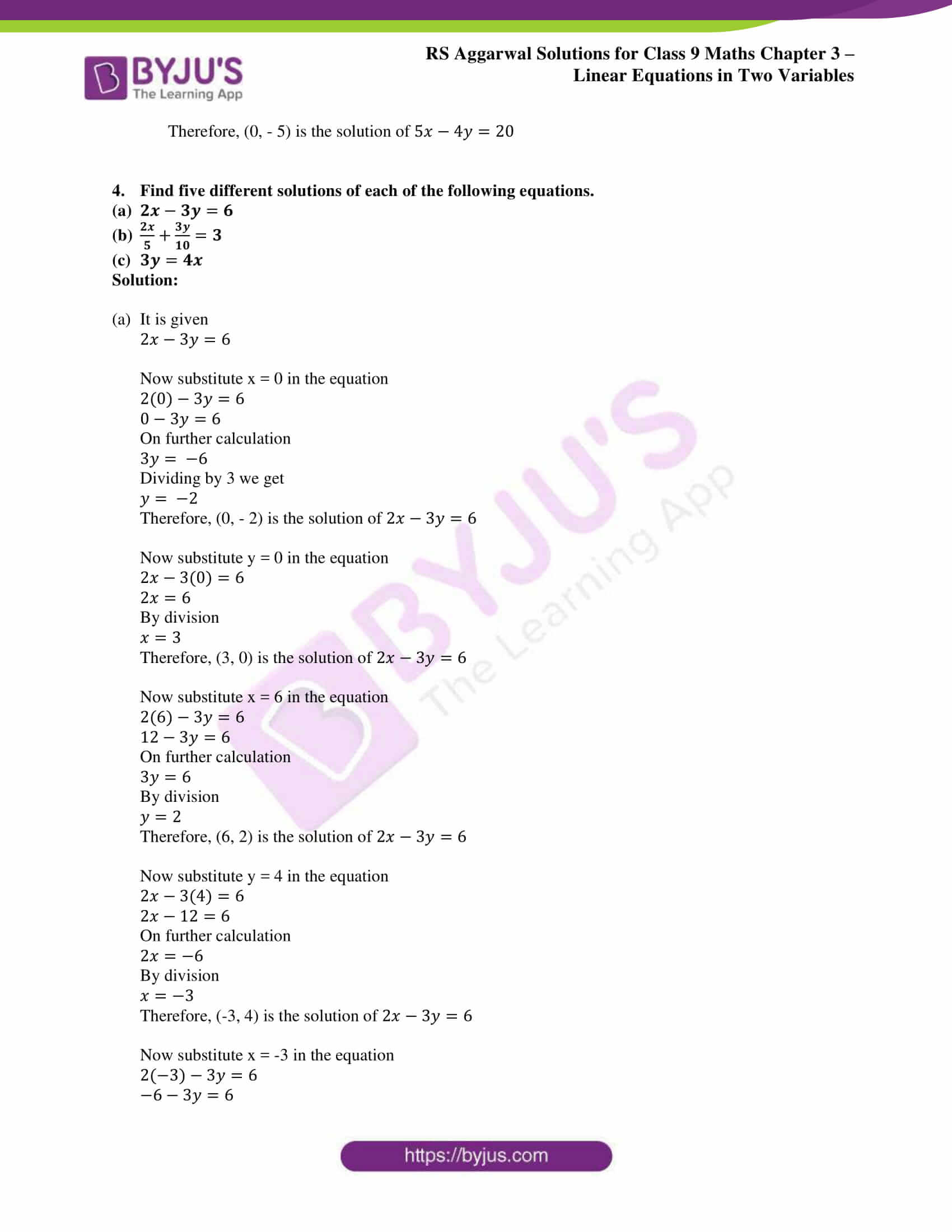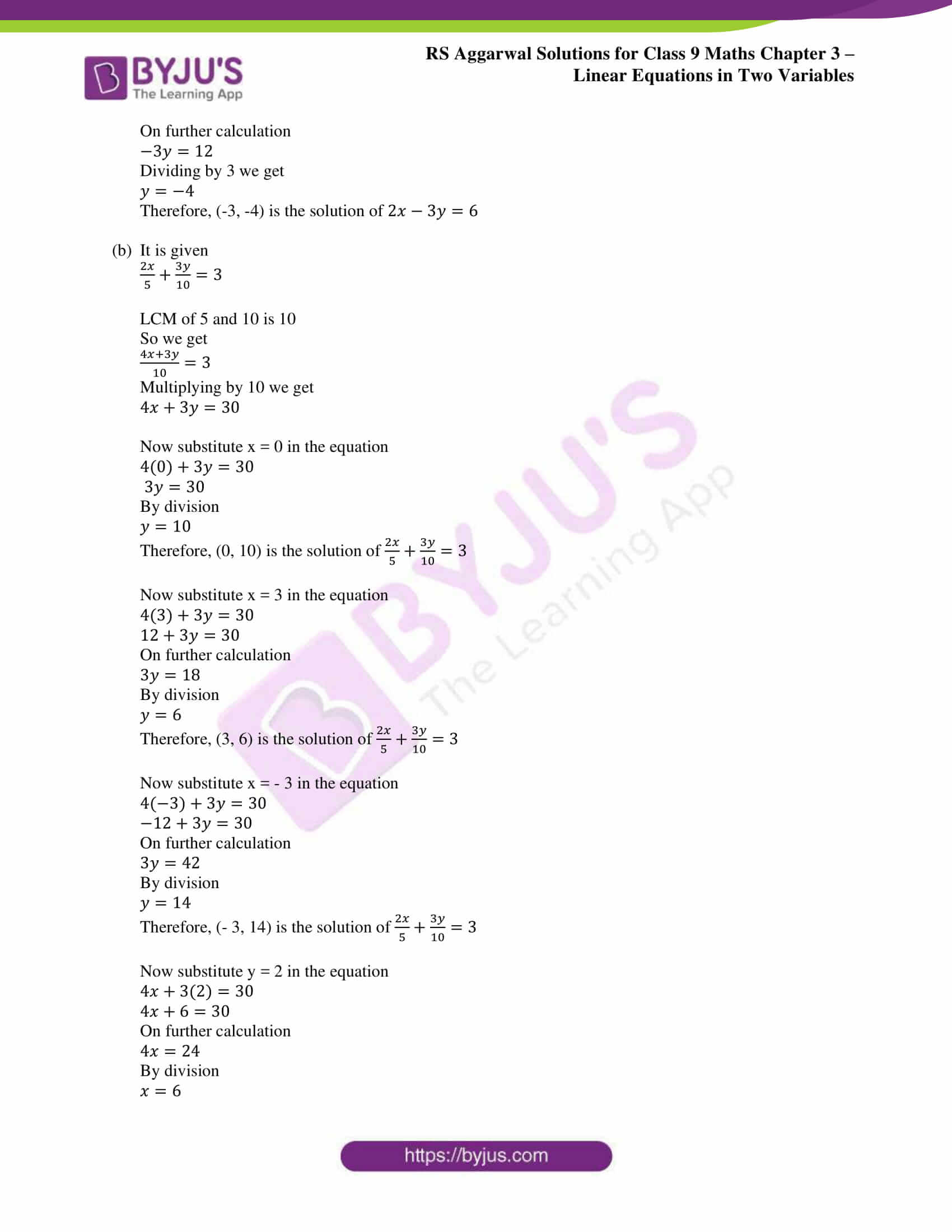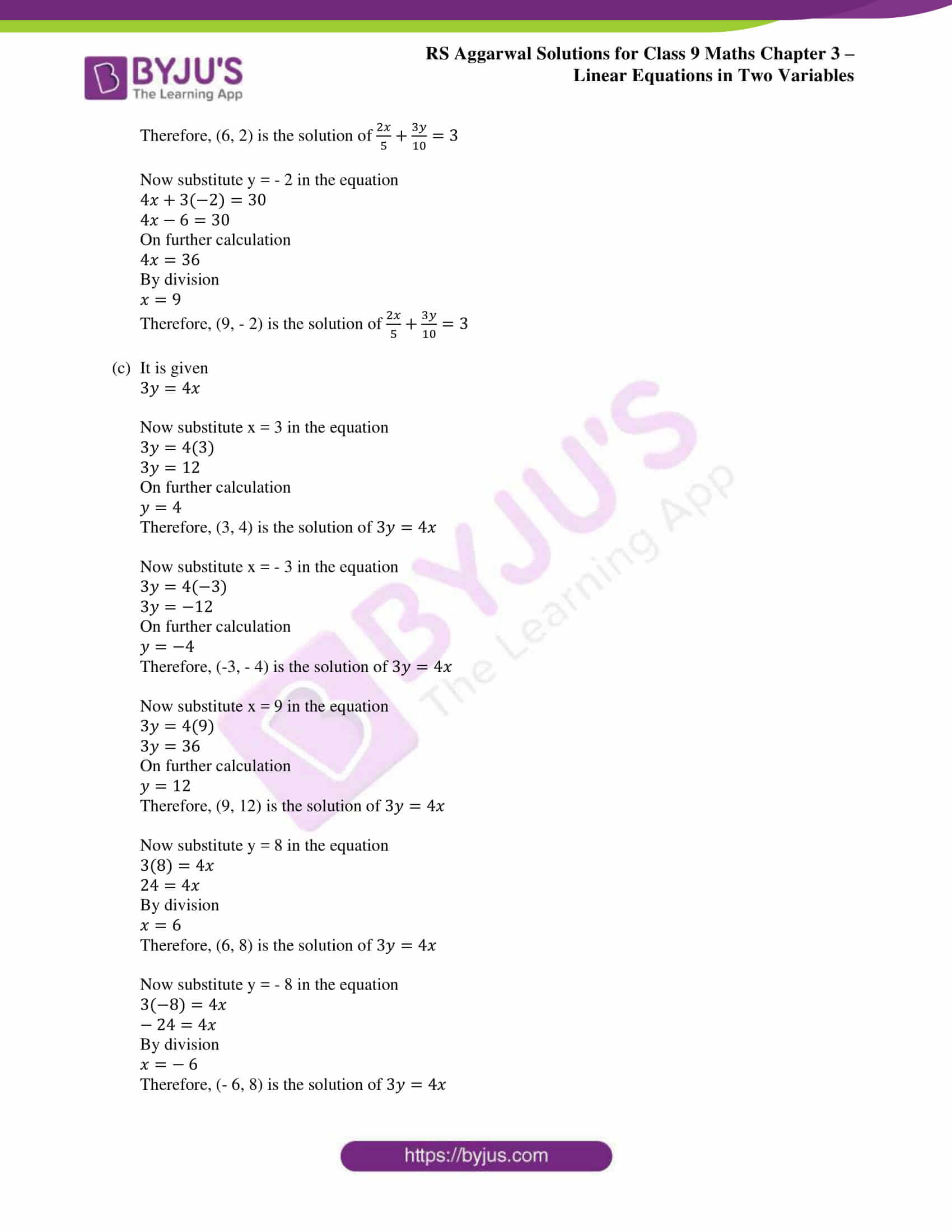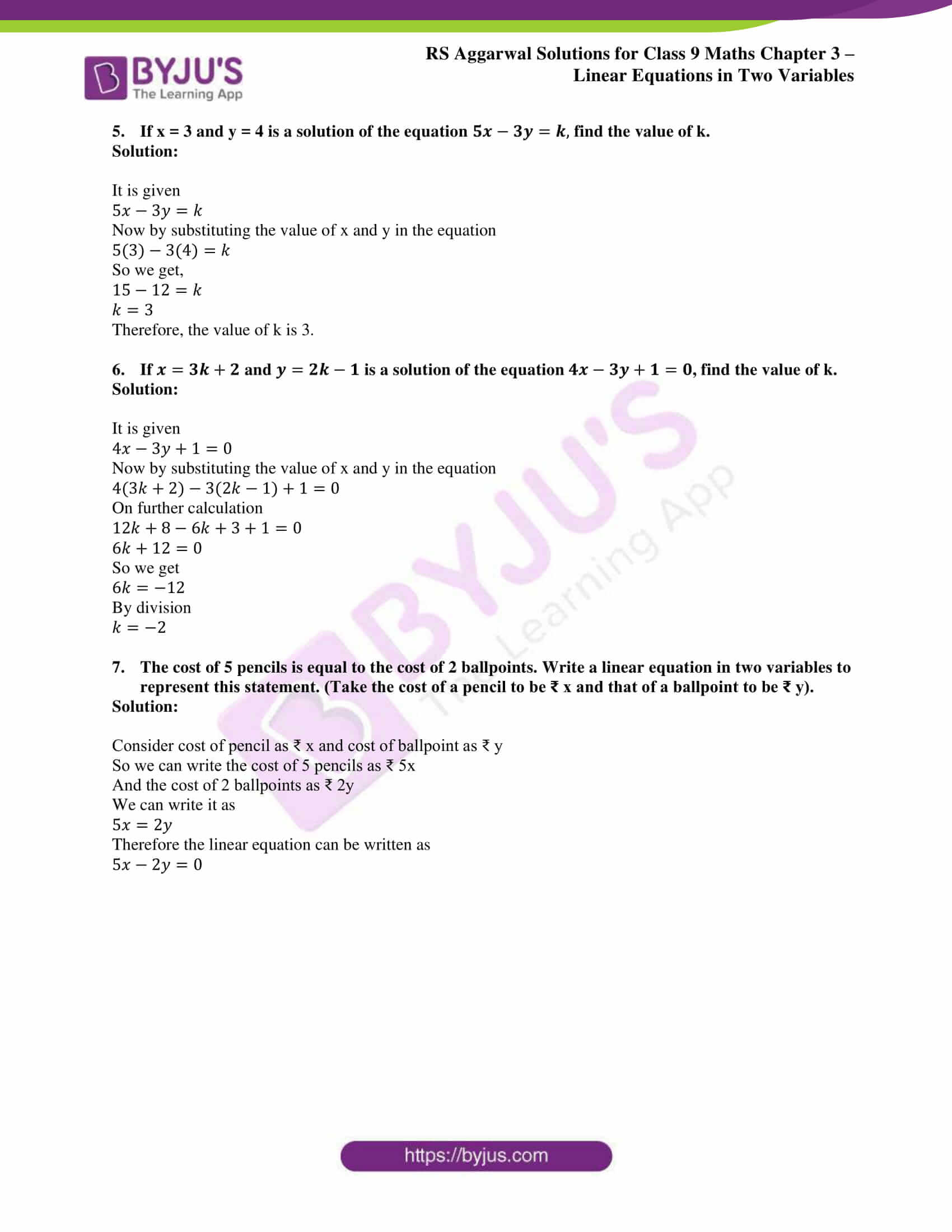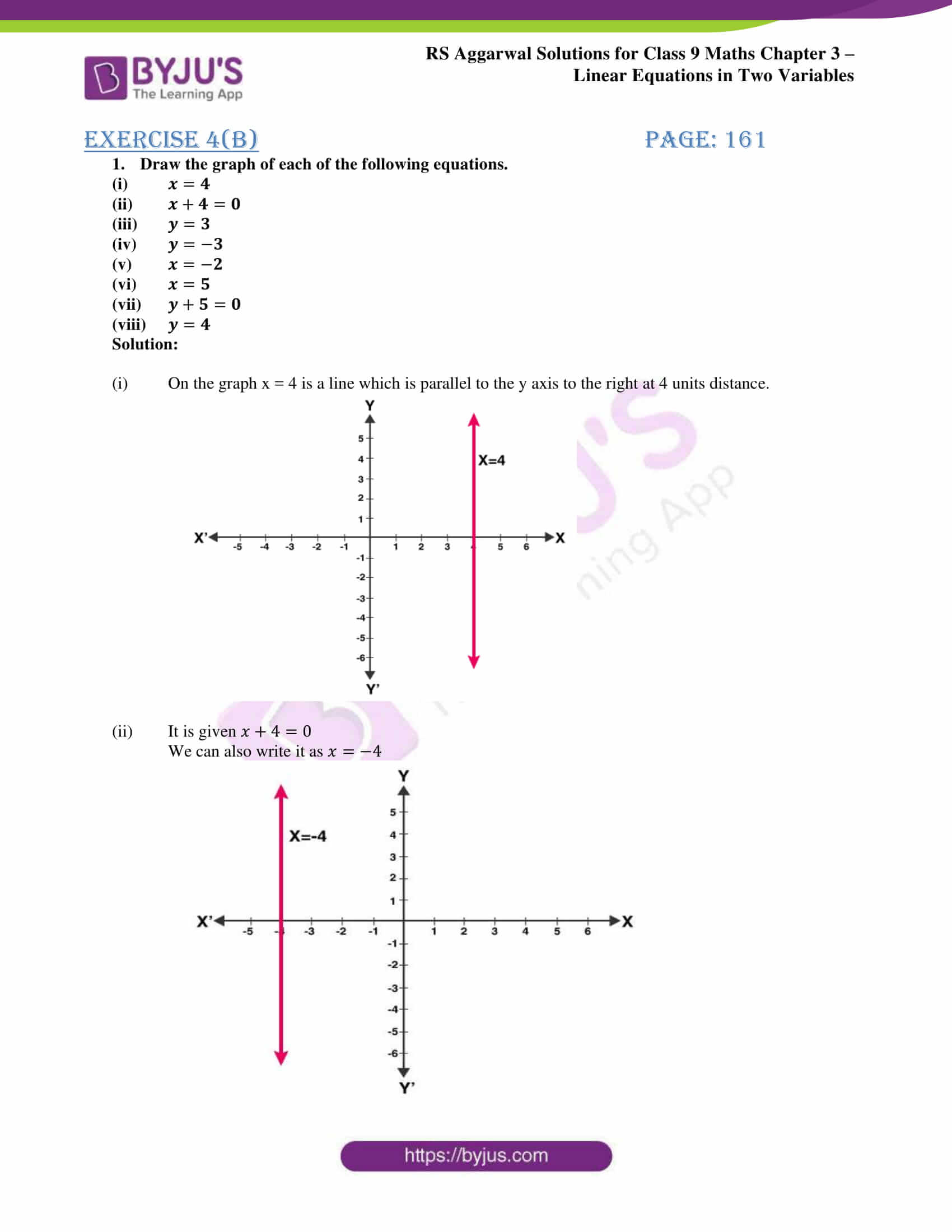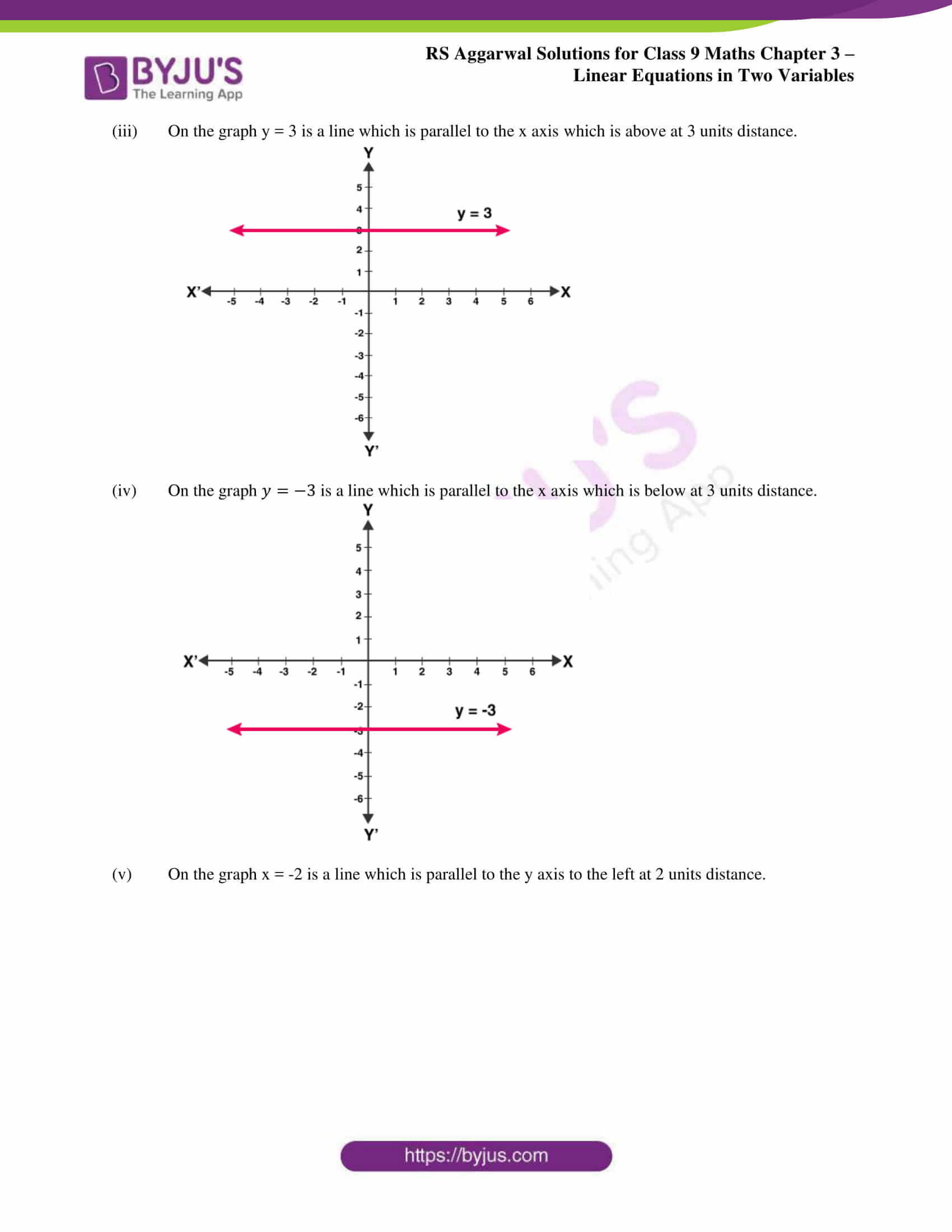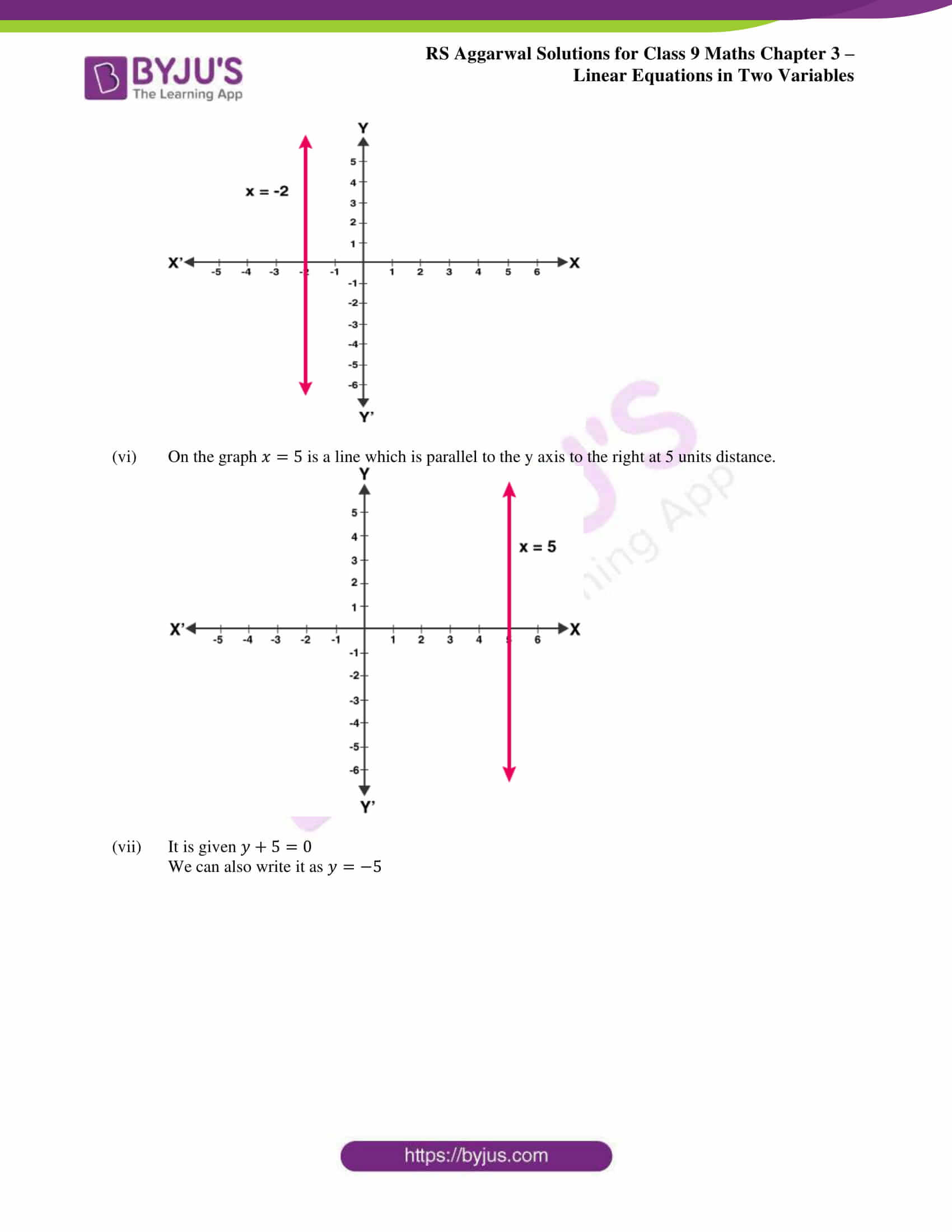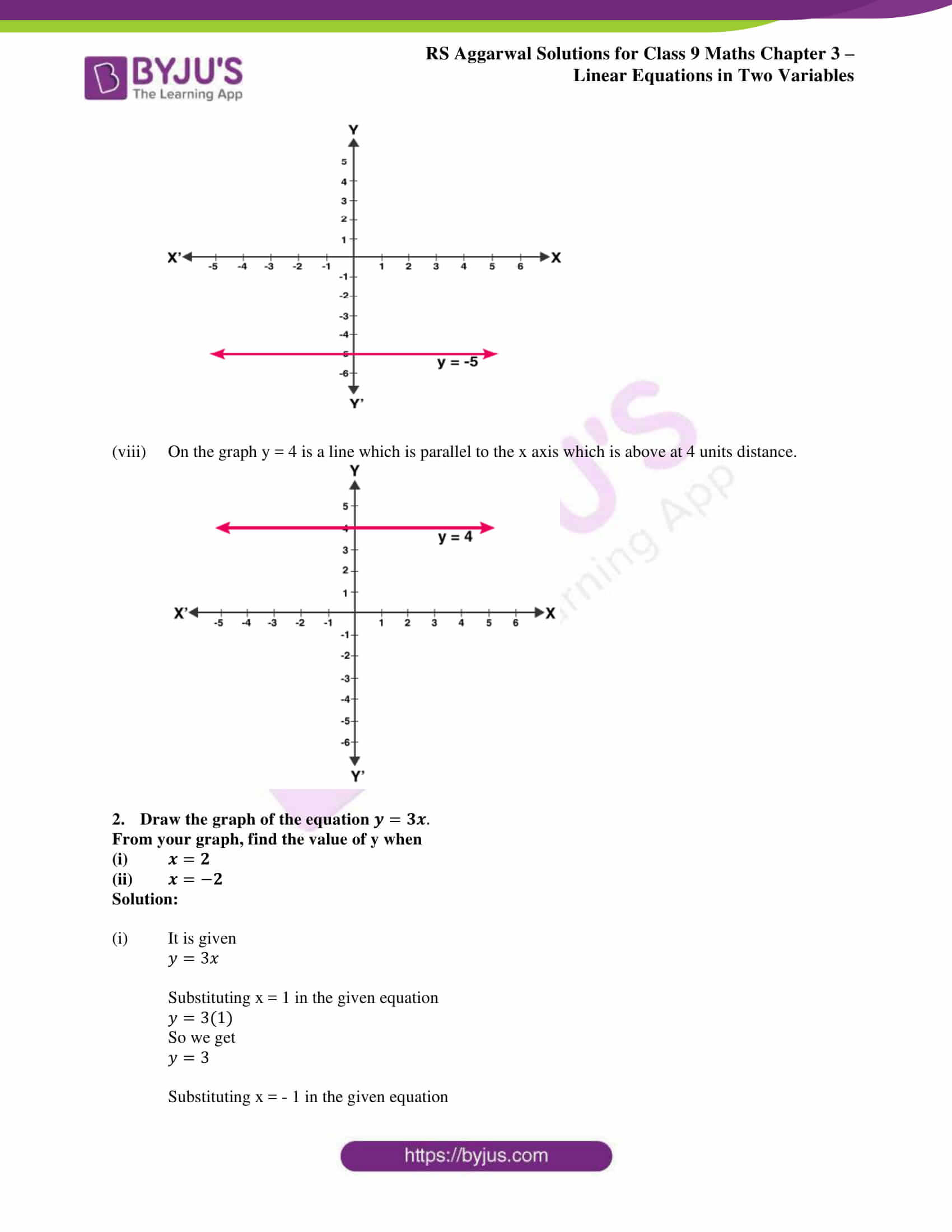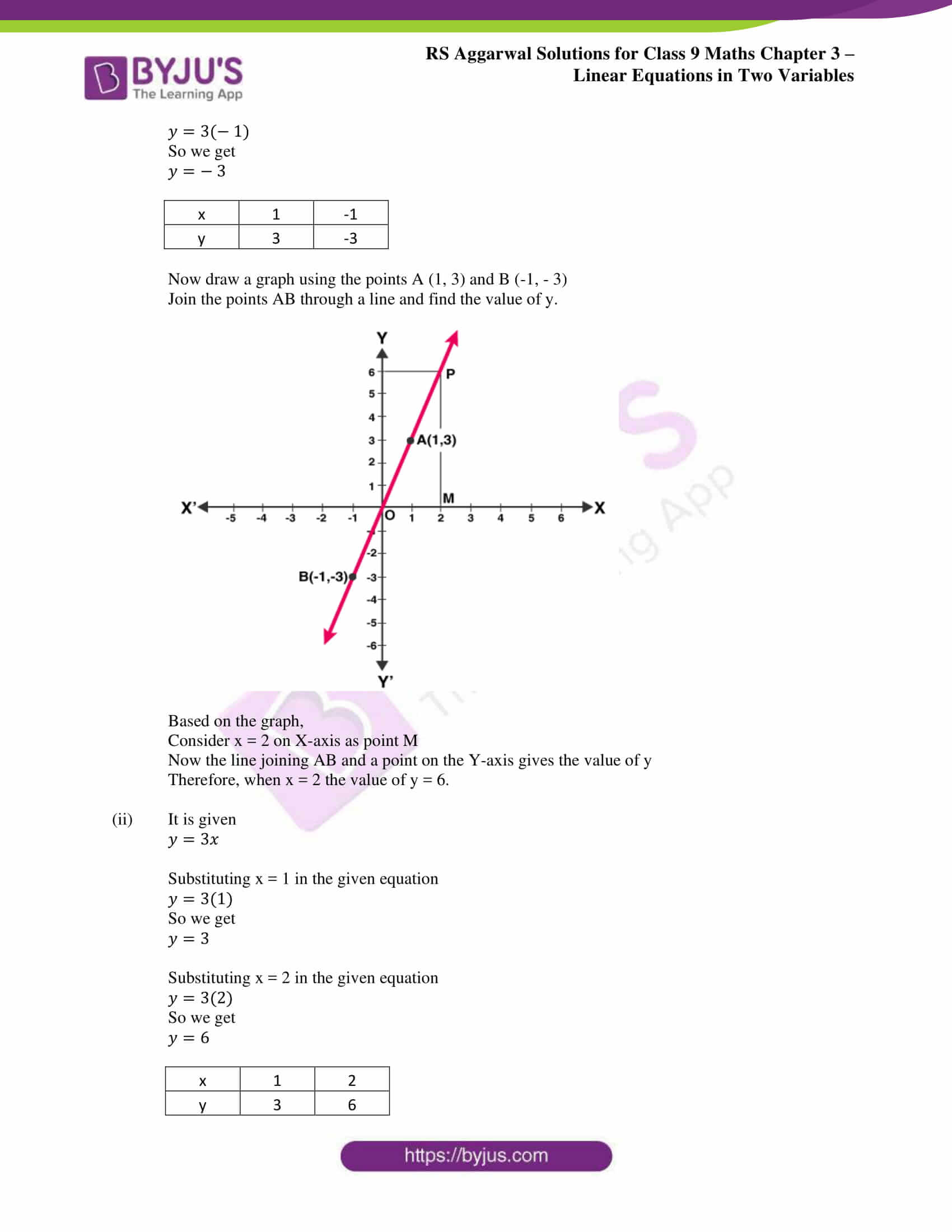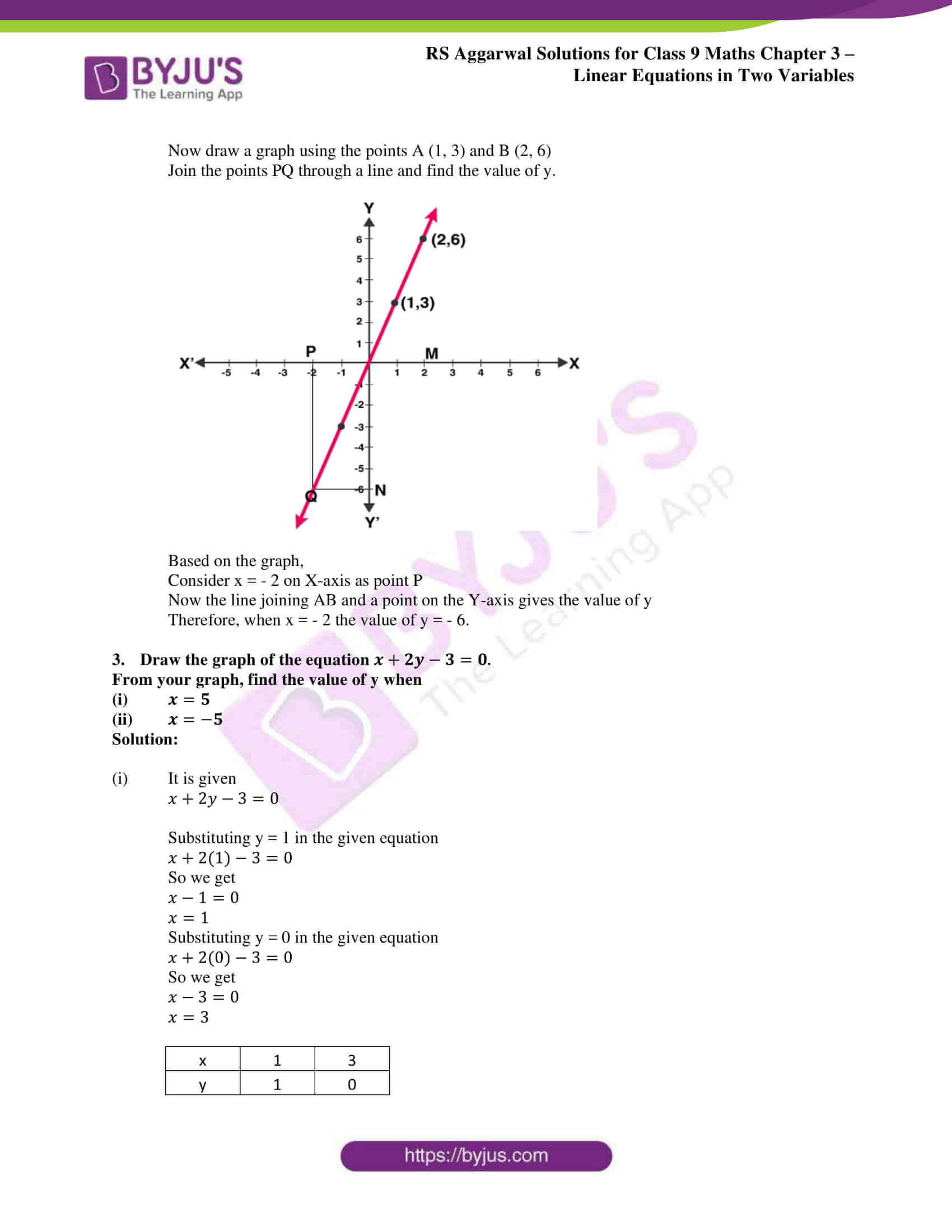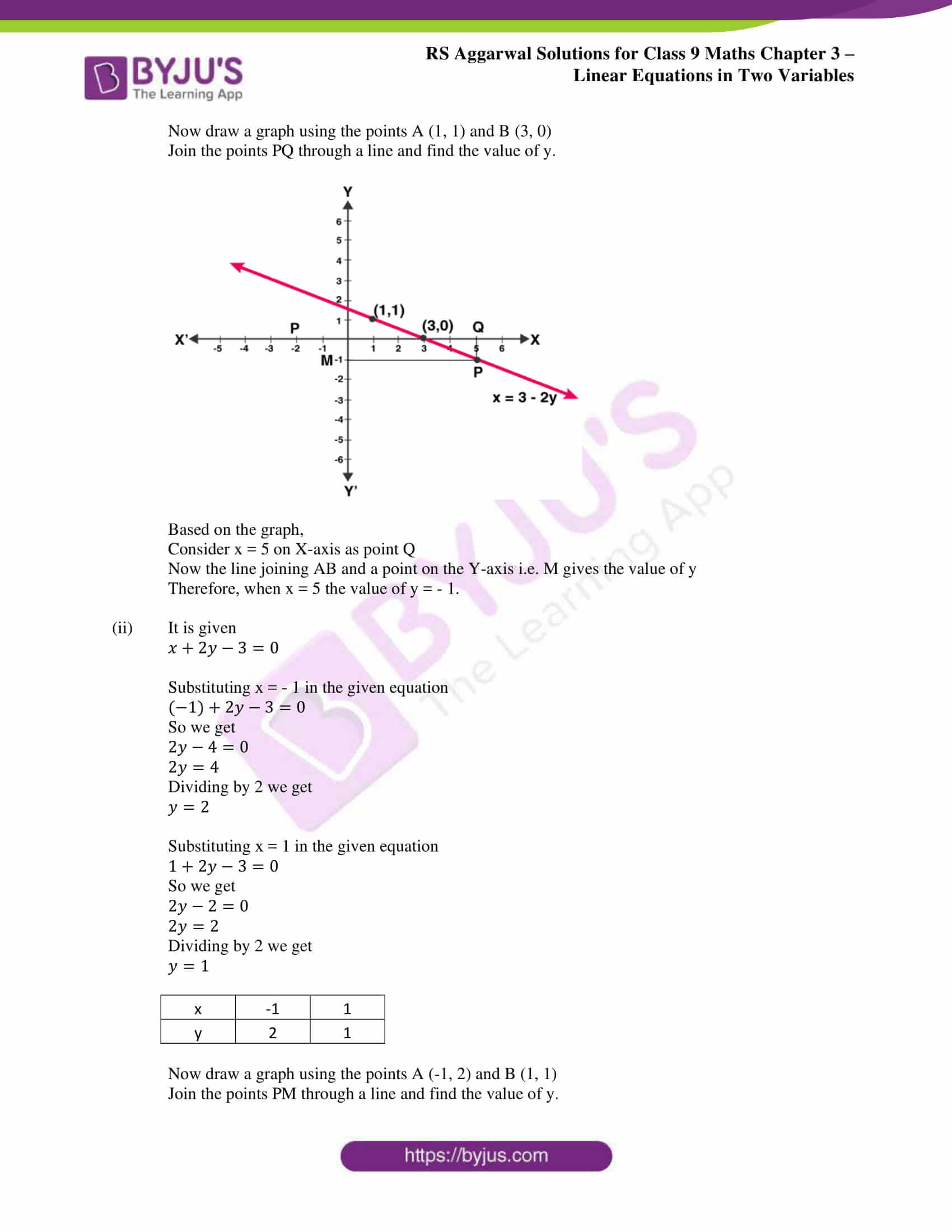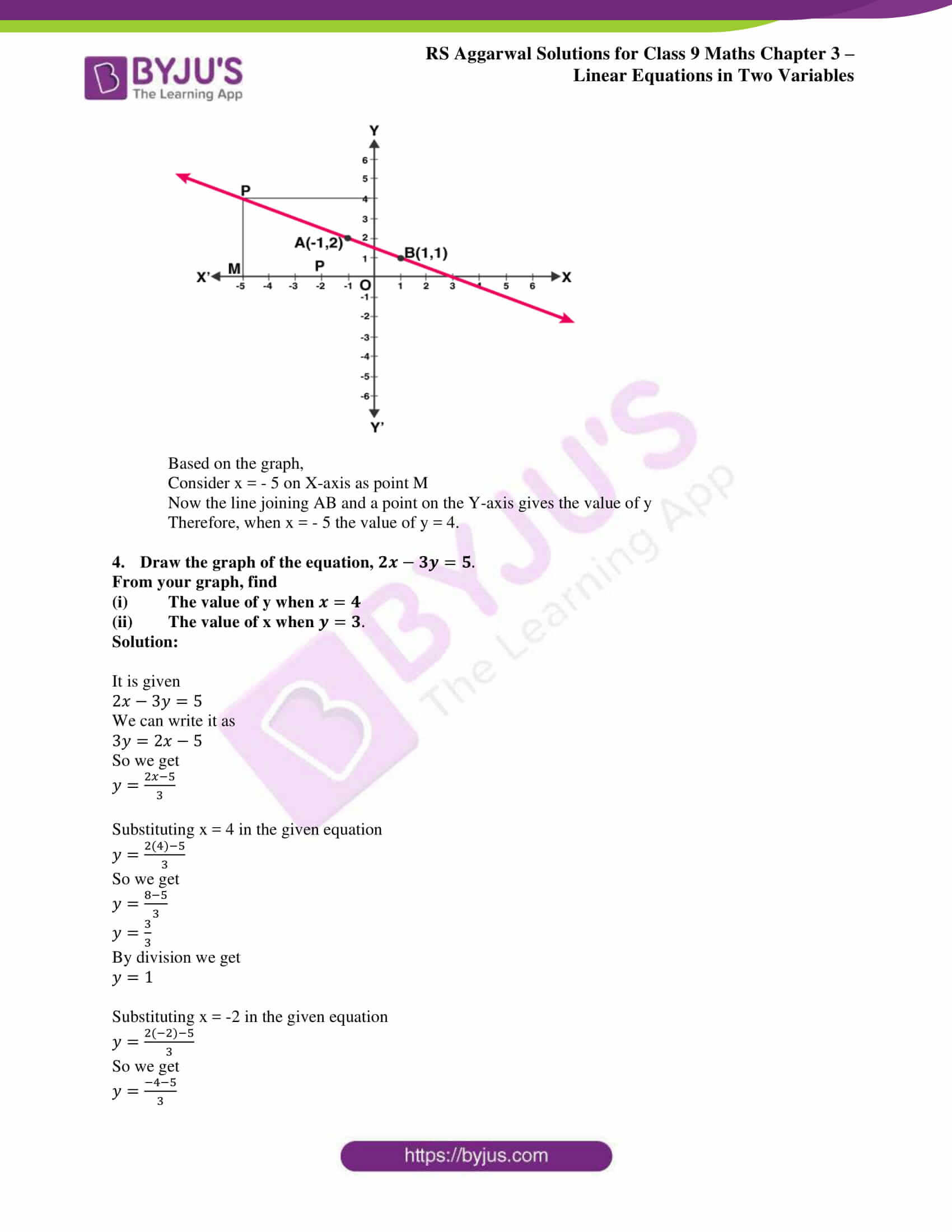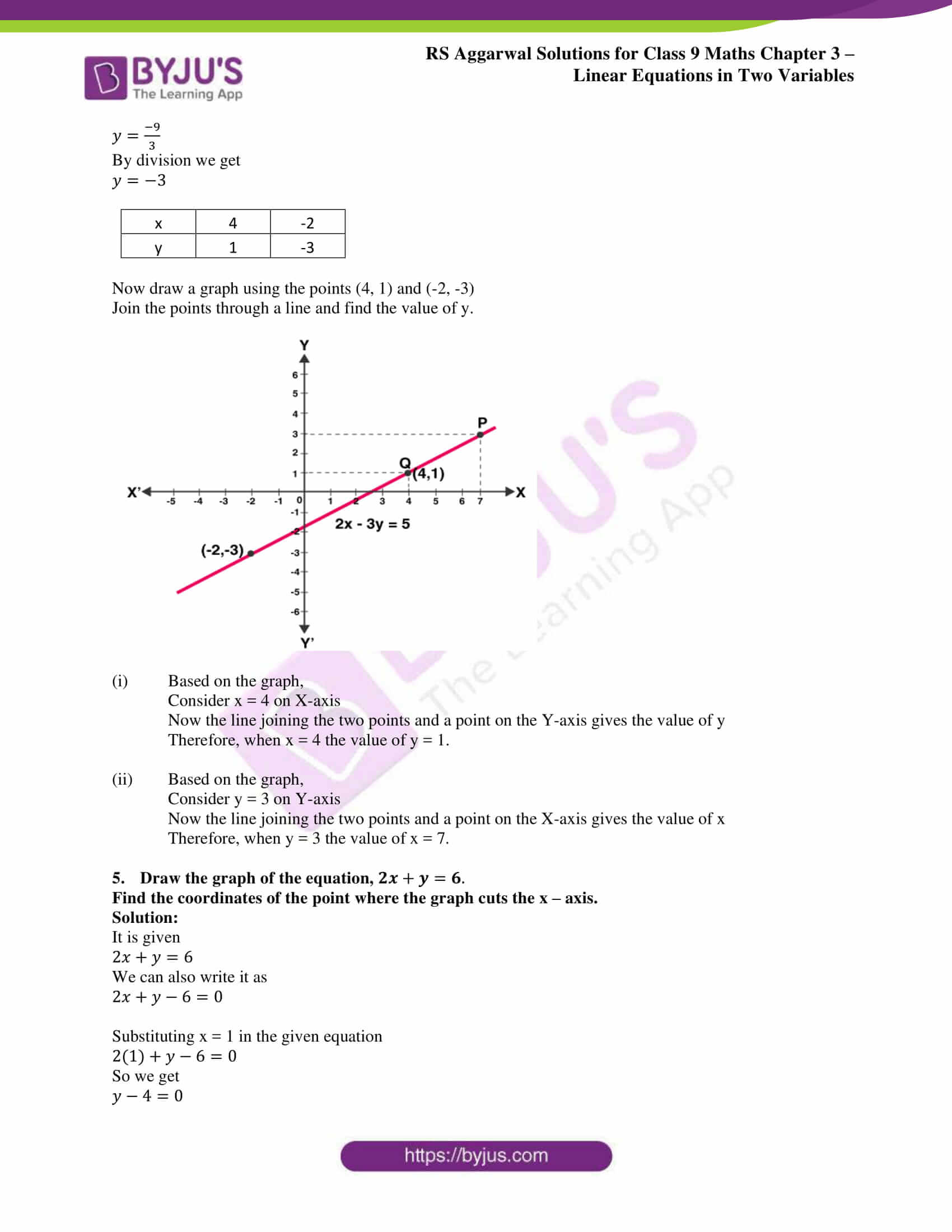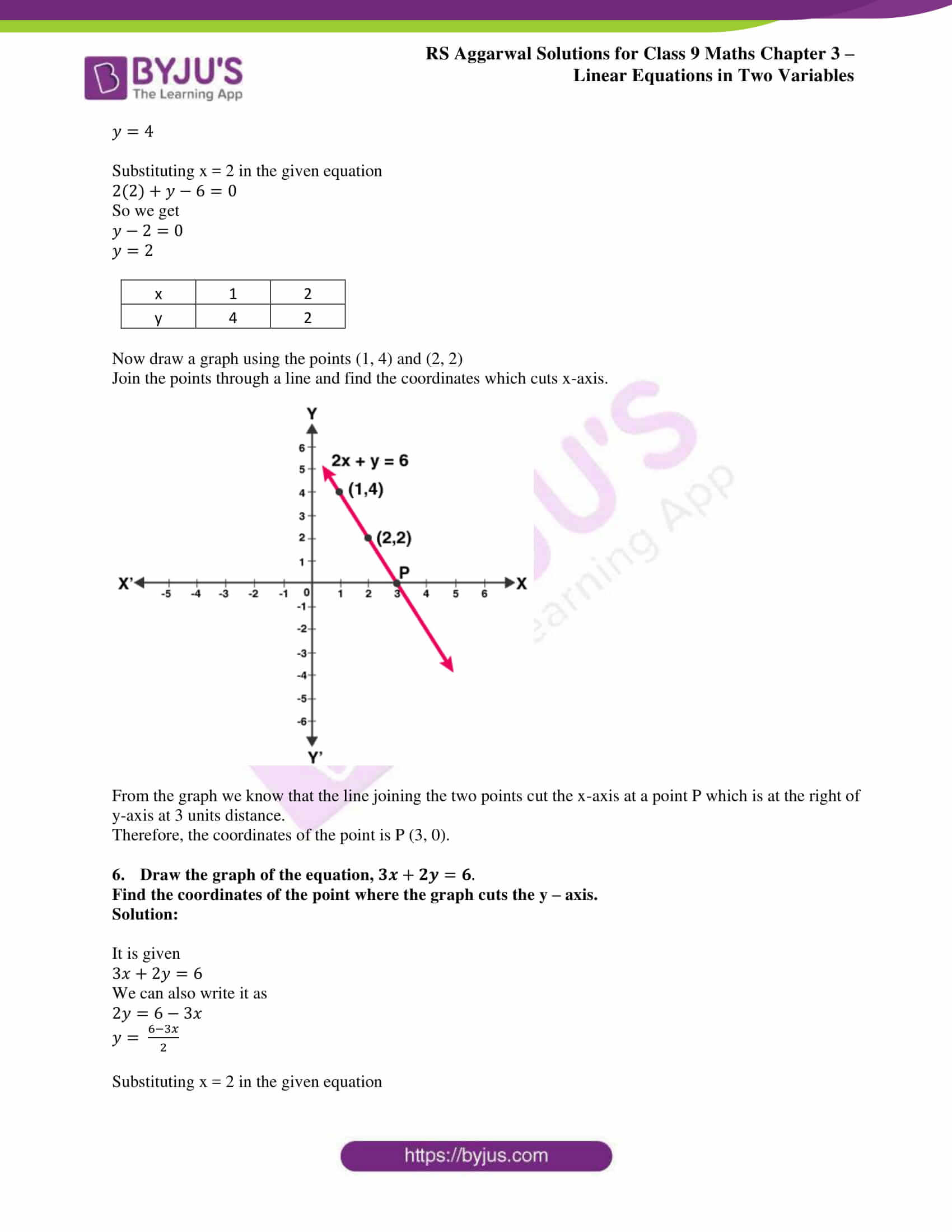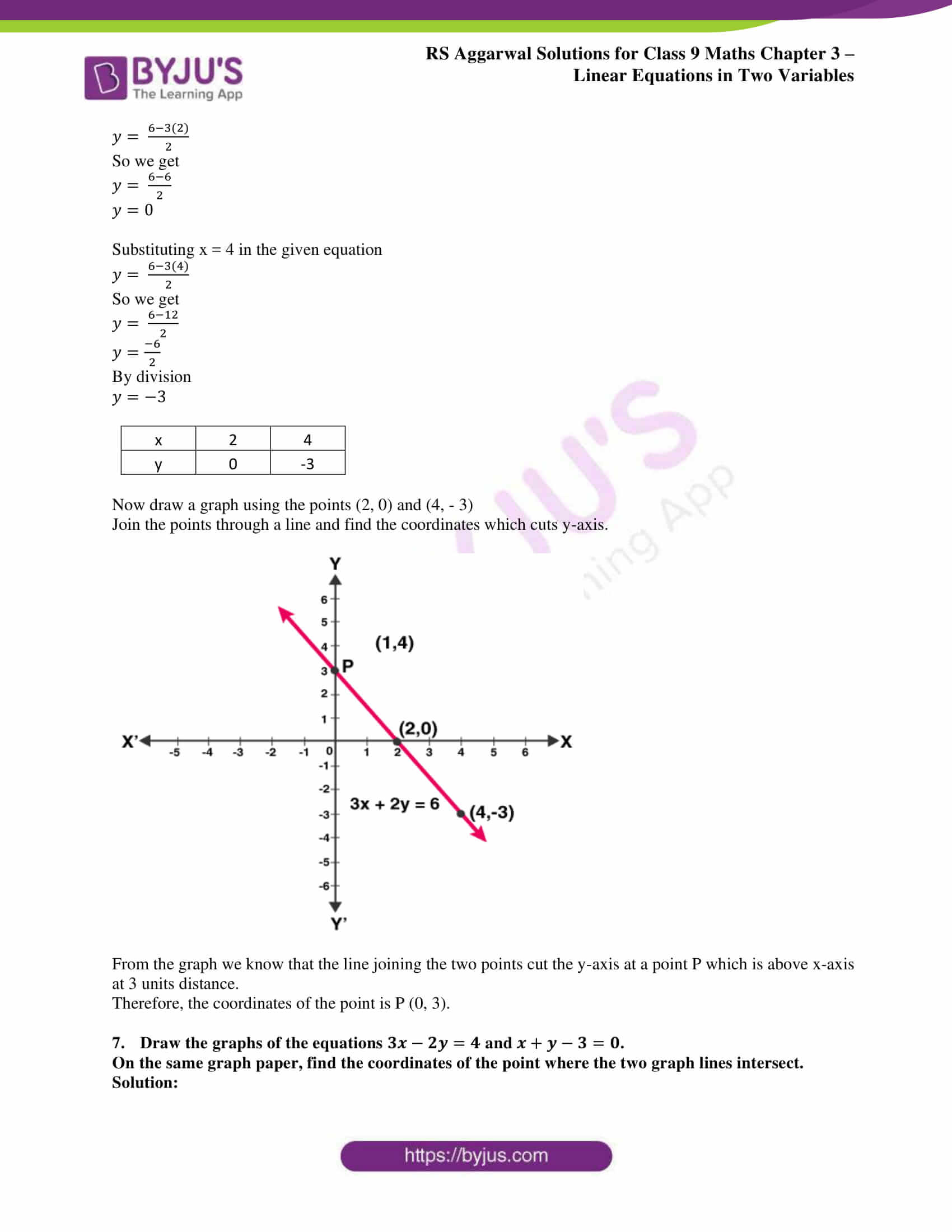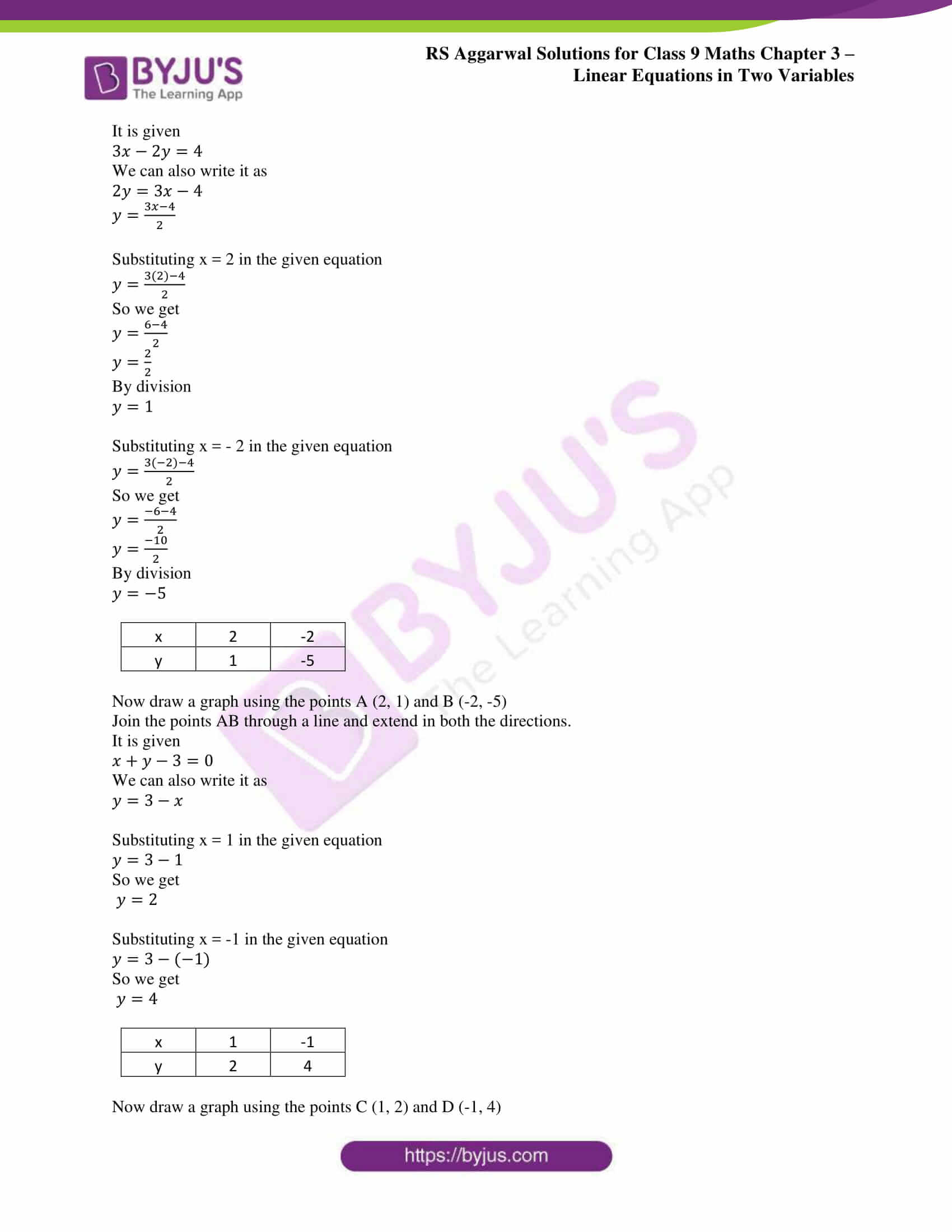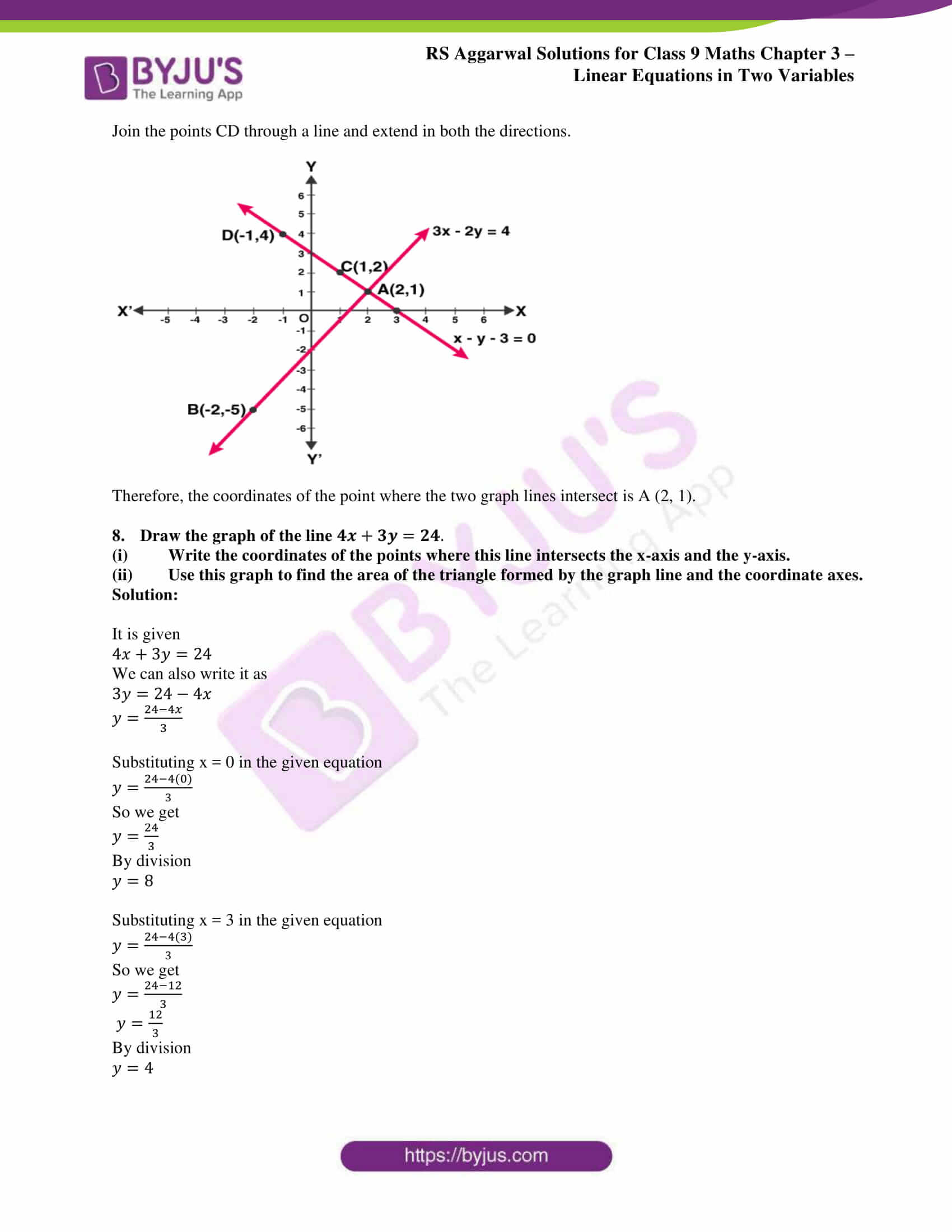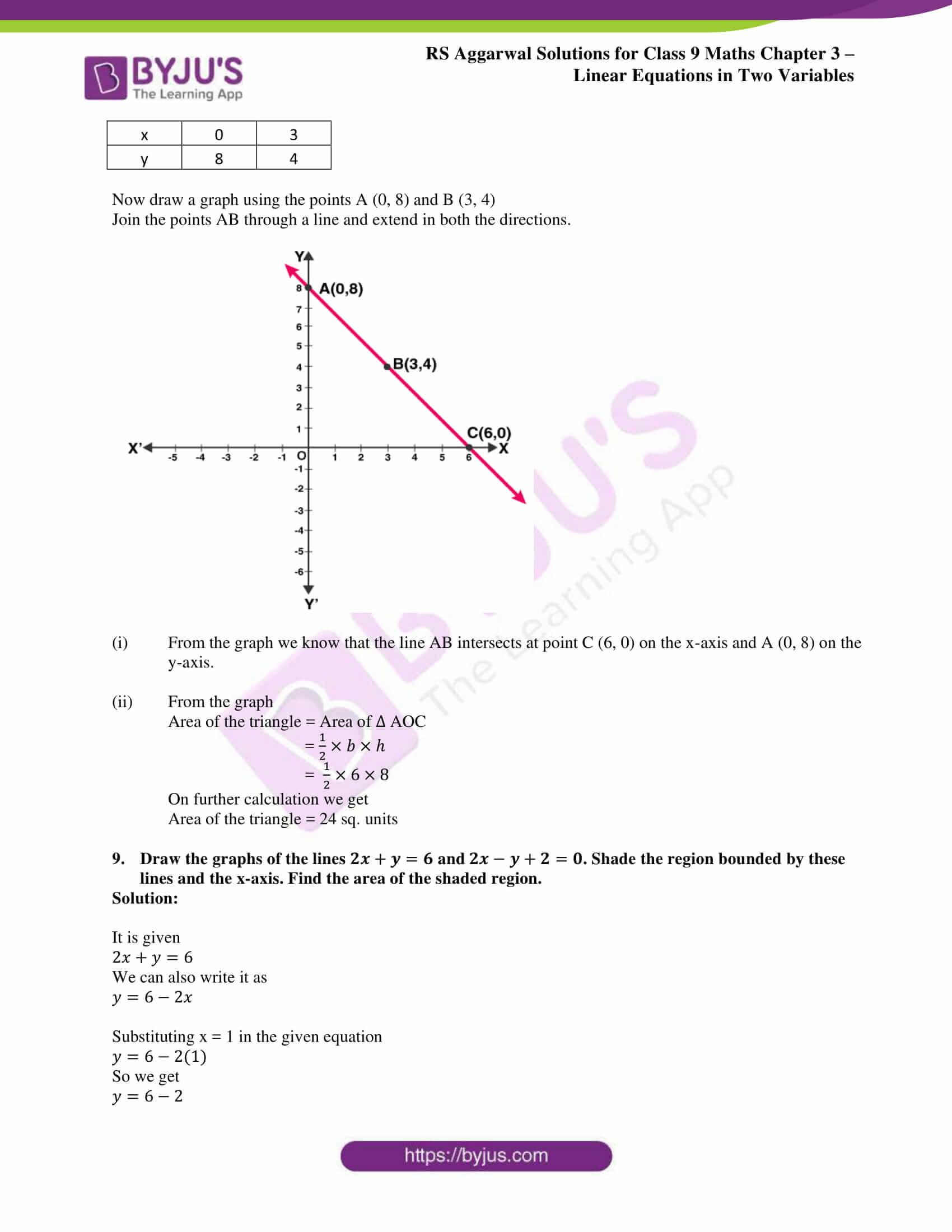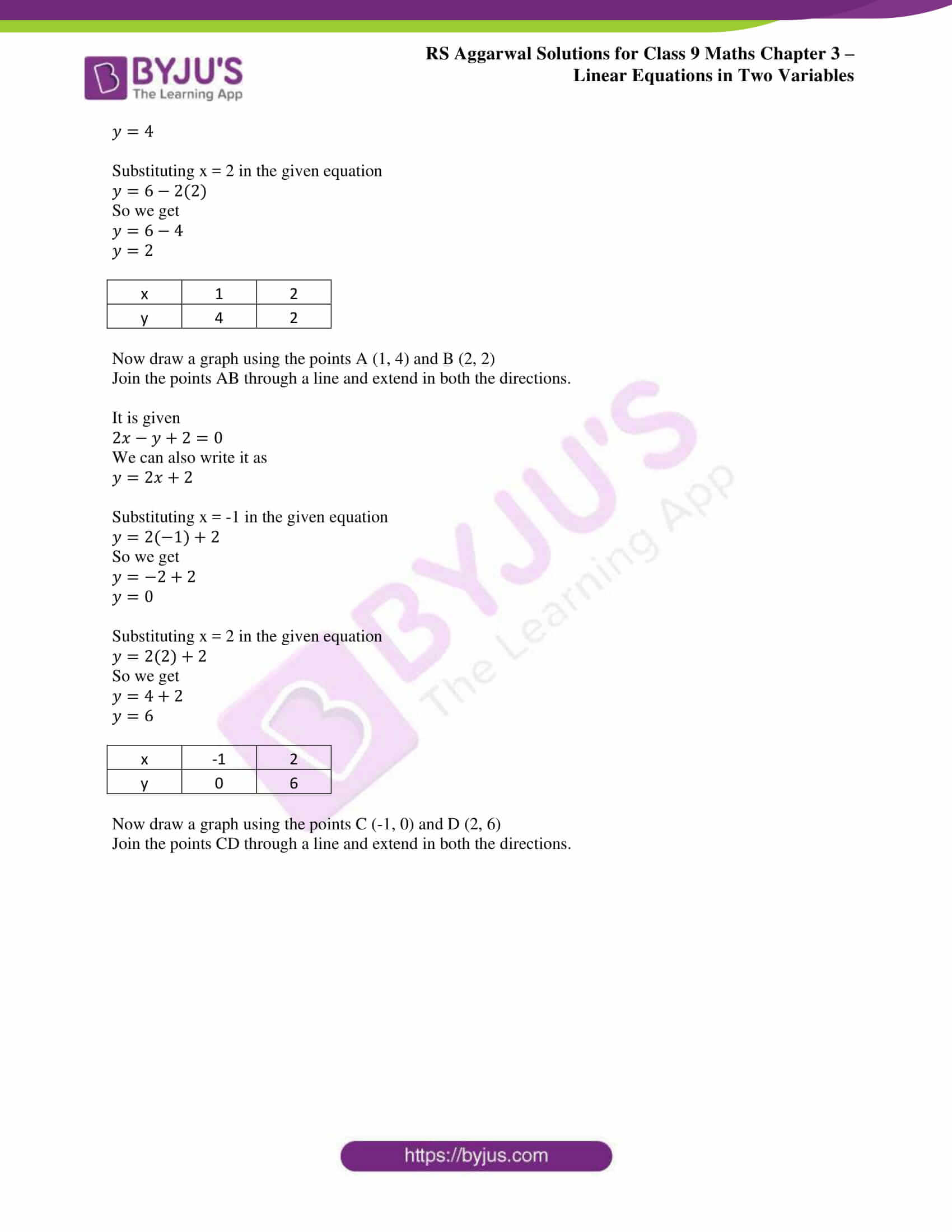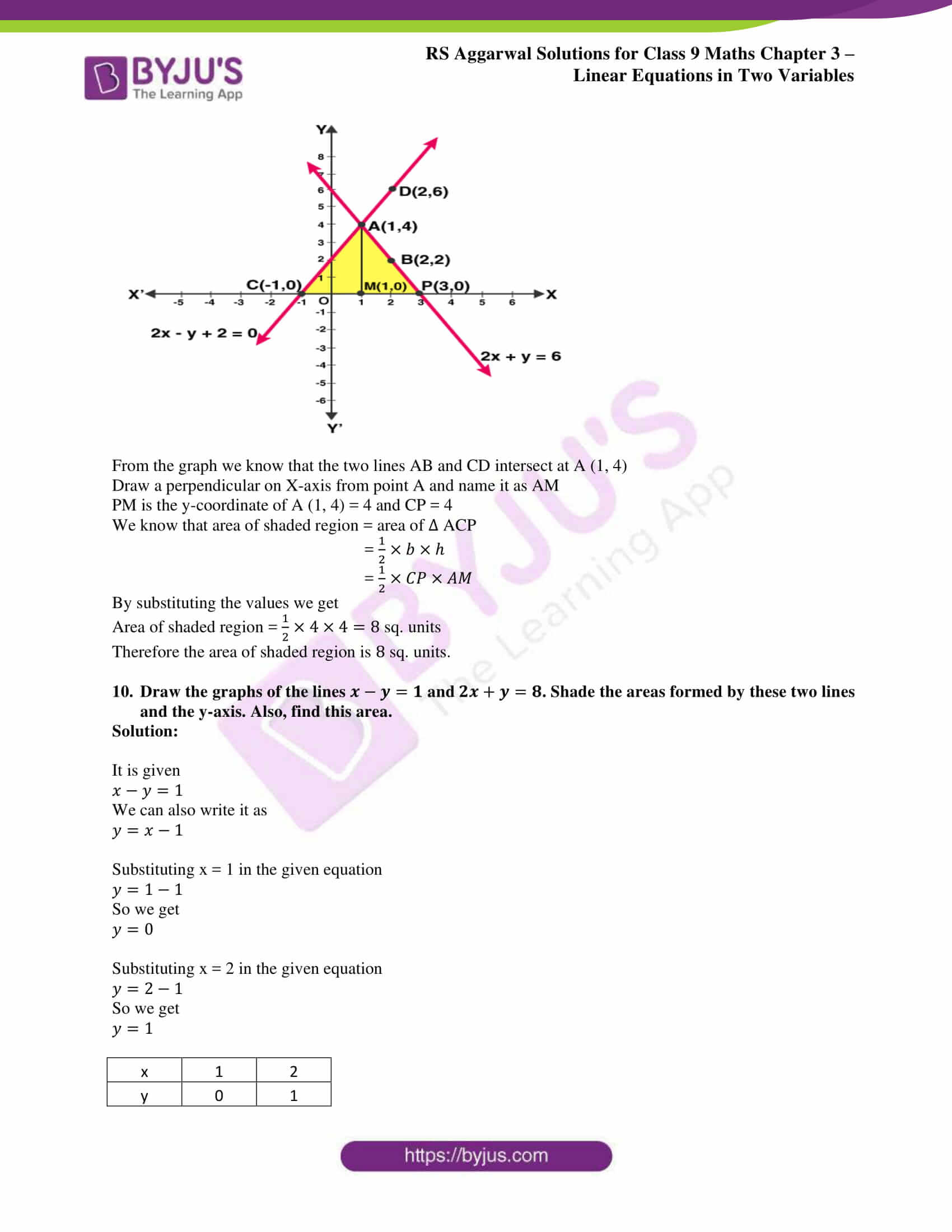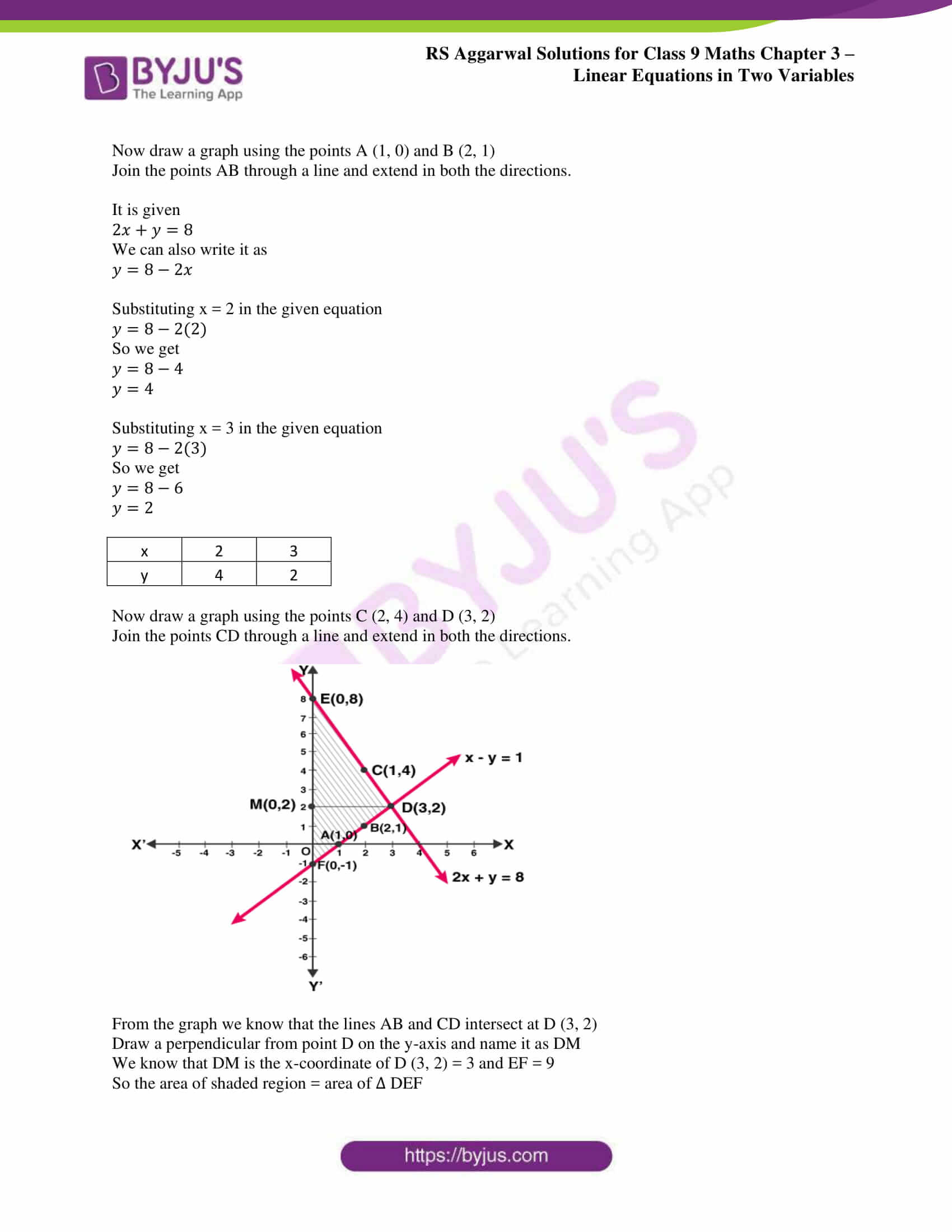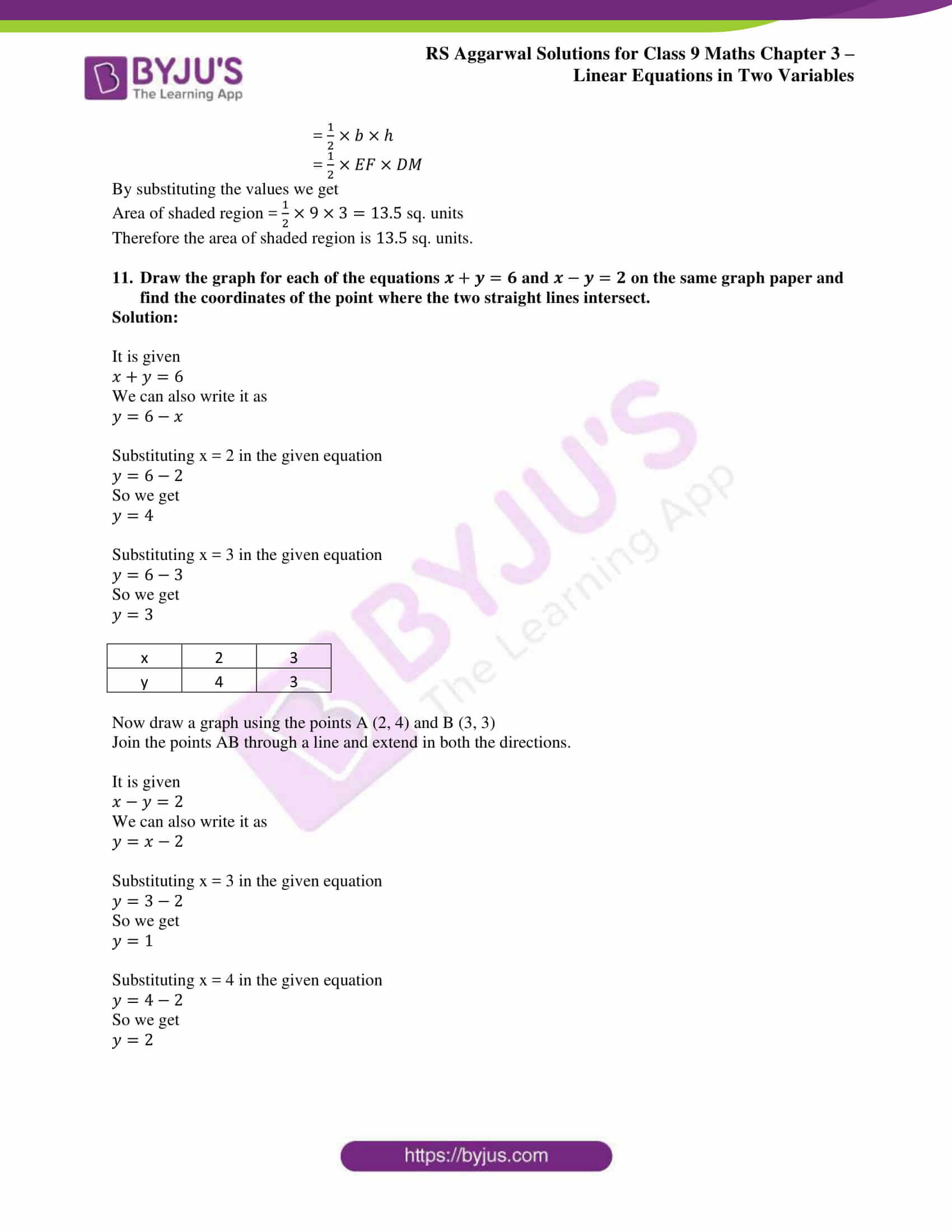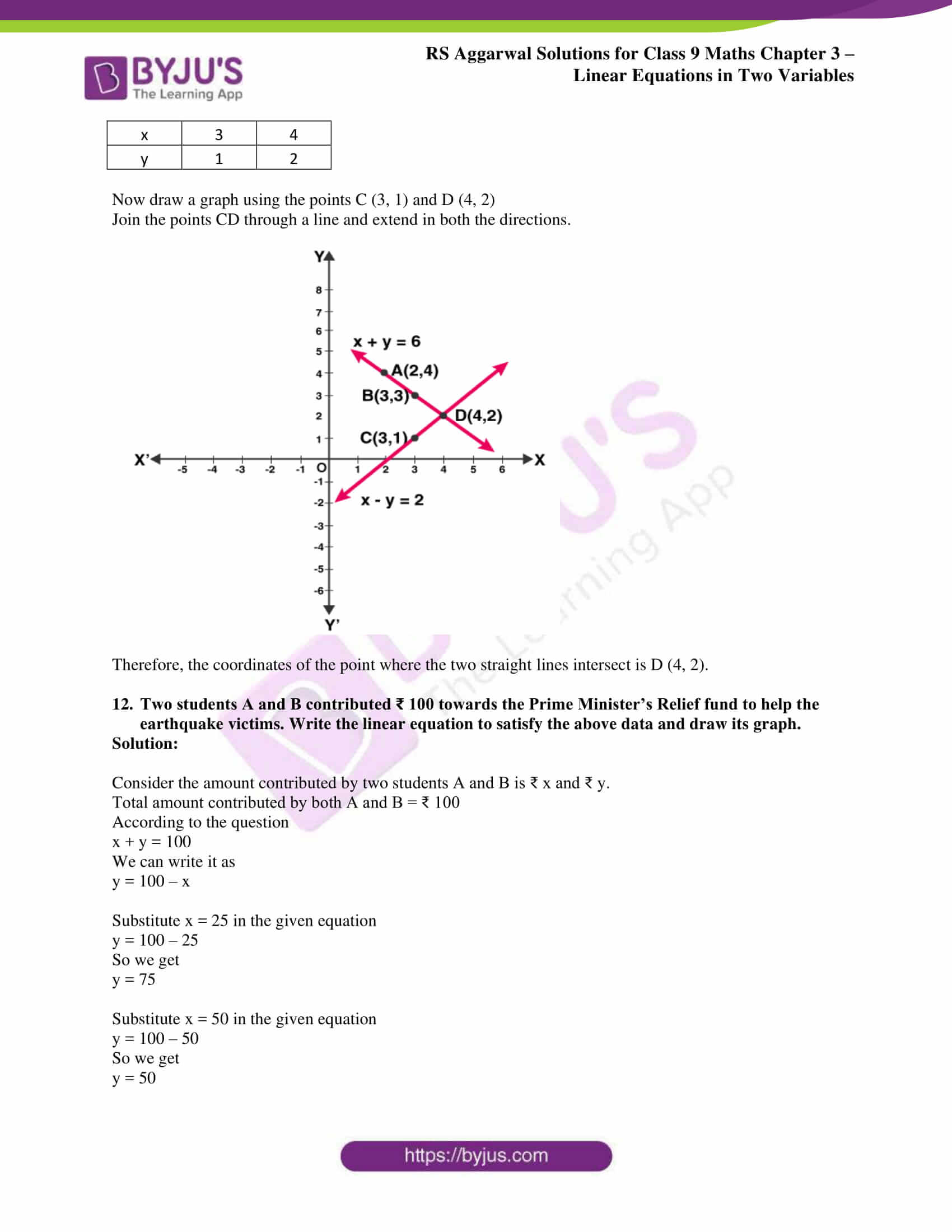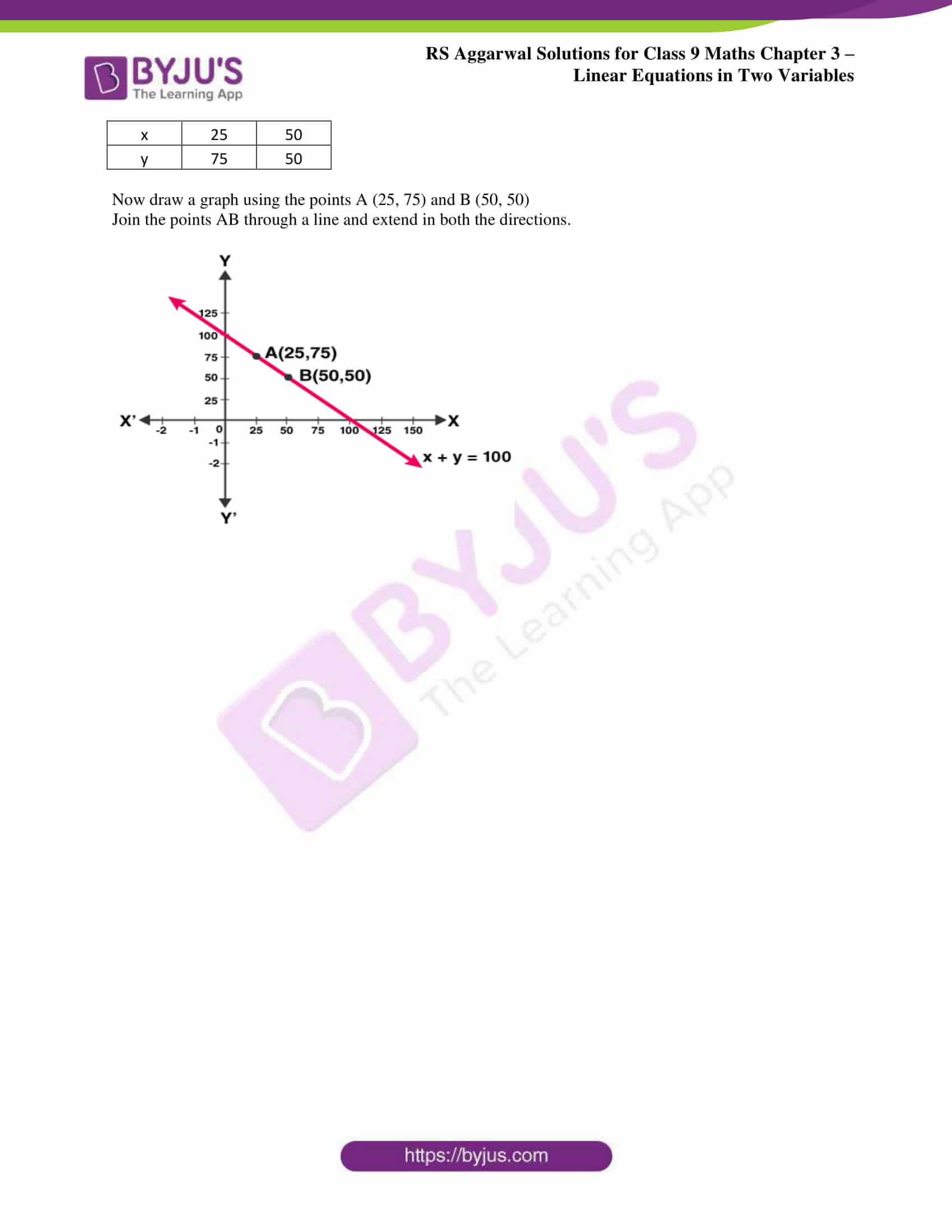### RS Aggarwal Solutions for Class 9 Maths Chapter 4: Linear Equations in Two Variables

The Chapter 4, Linear Equations in Two Variables, has 2 exercises and the RS Aggarwal Solutions provides the students with brief solutions to all the exercises as per the evaluation method designed by the education board. Chapter 4 includes various topics like:

• Introduction
• Linear Equations in Two Variables
• Solution of a linear equation
• Graph of linear equation in two variables

### RS Aggarwal Solutions Class 9 Maths Chapter 4 – Linear Equations in Two Variables

Students solving exercises can make use of RS Aggarwal Solutions for Class 9 as a reference in order to improve their confidence level to perform better in the exams. The students need to practice on a daily basis to have a good hold on the subject and solve the questions within the time constraints.

These solutions can also be referred by the students for last minute exam preparation to understand the steps and different methods used to solve the problems. Linear Equations in Two Variables has uses in various fields like variable costs, rates, budgeting and making predictions.